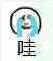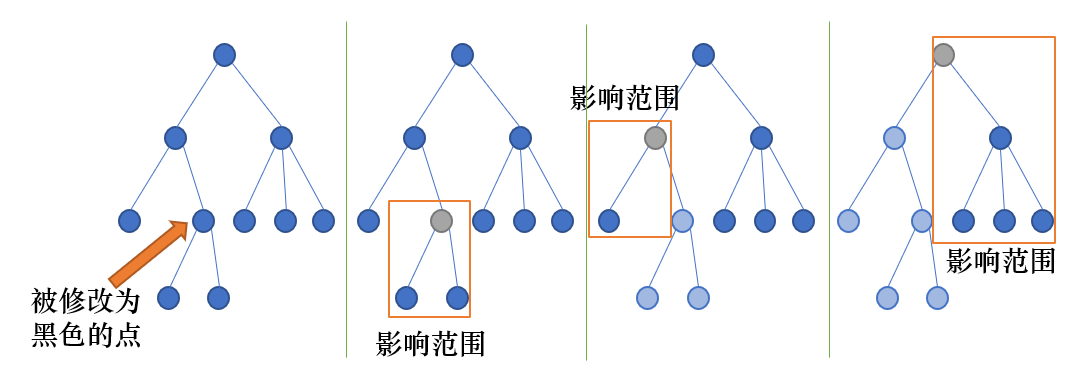# 最近公共祖先 题解【dfs序】【线段树】

煞笔题考场上没看出来……打了跟正解很接近的暴力…## 题目描述

• Modify v：将节点$v$的颜色改成黑色；
• Query v：找到一个黑色节点$u$，使得节点$u$和$v$的最近公共祖先$z$对应的权值尽可能大，输出节点$z$的权值。如果不存在黑色节点，输出$-1$。

## 输入输出样例

7 7
4 3 5 7 6 5 2
1 4
2 1
7 5
6 2
2 5
3 4
Query 1
Modify 2
Modify 4
Query 3
Modify 2
Modify 5
Query 6

-1
7
4


## 题解：

考试的时候以为暴力跳复杂度是错的，于是按着$20\%$小范围+$20\%$随机树，然后傻乎乎地全部暴力跳祖先了……随机树的点还挂了。下来发现暴力跳+特判，每个点最多更新两次就好了，后悔没好好想。

考虑既然LCA是祖先，那么两个点LCA的位置一定是到根节点的链上，考虑询问时在链上统计答案，但是这样统计答案无论如何都会做到$O(n^2)$。那么我们试着把问题转化为修改时统计答案，可以在把一个点变成黑色时更新它所能影响到的点，也就是沿着祖先更新不在当前节点子树内的点，这些点和刚才改变的点的LCA就是当前节点，因此可以用线段树对一段dfs序，也就是一棵子树进行修改。如果我们修改橙色箭头指示的位置，我们就沿着它的父亲跳，图中灰色点表示当前回溯到的点，浅色点表示已经修改的点，已经修改过的子树限制条件要多一些，因为被修改的点在这棵子树中，这些点互相之间的LCA也一定在这棵子树中。这时修改的就是两个区间了，也就是一个大区间挖去了中间一小部分，还是可以用线段树维护的。

于是考场上就打了这样一个算法……树的结构随机的部分分还没拿到。

这样一个算法已经很接近正解了。

因为每个节点会从某一个儿子更新上来，此时可以更新除了这个儿子以外的所有儿子。那么下一次从另外一个儿子更新过来就可以更新这个儿子了，也就是说一个节点最多被更新两次。如果发现一个节点已经被更新过，而且是被同一个儿子更新的，那么就不用更新；如果不是同一个儿子，就可以更新这个节点，之后不再更新这个节点及其祖先即可。因为当前节点已经被更新过，所以它的祖先也被它更新过，上面当然也不用更新了。

因此每个位置都被更新最多两次，时间复杂度为$O(m\log n)$。

还有一种DP做法，可以拿离线的部分分。做法是从上到下进行树形DP，然后$f[x]$表示$x$子树外可能产生贡献的LCA权值的最大值。假设dfs到了$u$节点，正准备进入儿子$v$，如果除了$v$以外的位置有黑色点，那么$f[v]$就可以用$w[u]$和$f[u]$中的最大值来更新。形式化的表述就是

$f[v]\left\{\begin{matrix} \max(f[u],w[u]) ,& \exists Blackpoints\ \text{in}\ subtree(x)-subtree(y) \\ f[u] ,& \not\exists Blackpoints\ \text{in}\ subtree(x)-subtree(y)\end{matrix}\right.$

代码是正解代码。

## Code：

#include<cstdio>
#include<cstring>
#include<algorithm>
#define ls (k<<1)
#define rs (k<<1|1)
#define mid ((l+r)>>1)
using std::max;
struct node
{
int l,r,v,lazy;
node(int l,int r)
{
this->l=l;
this->r=r;
v=-1;
lazy=0;
}
node(){}
}t;
void build(int k,int l,int r)
{
t[k]=node(l,r);
if(l==r)
return;
build(ls,l,mid);
build(rs,mid+1,r);
}
void pushdown(int k)
{
int x=t[k].lazy;
if(!x||t[k].l==t[k].r)
return;
t[k].lazy=0;
t[ls].lazy=max(t[ls].lazy,x);
t[ls].v=max(t[ls].v,x);
t[rs].lazy=max(t[rs].lazy,x);
t[rs].v=max(t[rs].v,x);
}
void change(int k,int l,int r,int x)
{
if(r<t[k].l||l>t[k].r)
return;
if(l<=t[k].l&&r>=t[k].r)
{
t[k].lazy=max(t[k].lazy,x);
t[k].v=max(t[k].v,x);
return;
}
pushdown(k);
change(ls,l,r,x);
change(rs,l,r,x);
t[k].v=max(t[ls].v,t[rs].v);
}
{
if(p<t[k].l||p>t[k].r)
return -1;
if(t[k].l==t[k].r)
return t[k].v;
pushdown(k);
}
struct edge
{
int n,nxt;
edge(int n,int nxt)
{
this->n=n;
this->nxt=nxt;
}
edge(){}
}e;
{
}
int dfn,fa,sz,cnt=0;
void dfs(int x)
{
dfn[x]=++cnt;
sz[x]=1;
if(e[i].n!=fa[x])
{
fa[e[i].n]=x;
dfs(e[i].n);
sz[x]+=sz[e[i].n];
}
}
char op;
int w,from;//第一次从哪个点被找上来,为-1说明被找过两次了
int main()
{
int n,m,u,v;
scanf("%d%d",&n,&m);
build(1,1,n);
for(int i=1;i<=n;++i)
scanf("%d",&w[i]);
for(int i=1;i<n;++i)
{
scanf("%d%d",&u,&v);
}
fa=0;
dfs(1);
for(int i=1;i<=m;++i)
{
scanf("%s",op);
scanf("%d",&u);
if(op=='M')
{
int l=dfn[u];
int r=dfn[u]+sz[u]-1;
change(1,l,r,w[u]);
if(from[fa[u]]==-1||from[fa[u]]==u)
continue;
else if(from[fa[u]]==0)
from[fa[u]]=u;
else
from[fa[u]]=-1;
u=fa[u];
while(u!=0)
{
change(1,dfn[u],l-1,w[u]);
change(1,r+1,dfn[u]+sz[u]-1,w[u]);
if(from[fa[u]]==-1||from[fa[u]]==u)
break;
else if(from[fa[u]]==0)
from[fa[u]]=u;
else
from[fa[u]]=-1;
l=dfn[u];
r=dfn[u]+sz[u]-1;
u=fa[u];
}
}
else
}
return 0;
}

### 说点什么Subscribe

/* */